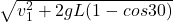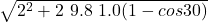## Fig 1 shows a pendulum of length L = 1.0 m. Its ball has speed of vo=2.0 m/s when the cord makes an angle of 30 degrees with the verti

Question

Fig 1 shows a pendulum of length L = 1.0 m. Its ball has speed of vo=2.0
m/s when the cord makes an angle of 30 degrees with the vertical. What
is the speed (V) of the ball when it passes the lowest position?

in progress 0
2 weeks 2021-09-05T13:46:50+00:00 1 Answers 0 views 0

v = 2.57 m / s

Explanation:

For this exercise let’s use conservation of energy

starting point. When it is at an angle of 30º

Em₀ = K + U = ½ m v₁² + m g y₁

final point. Lowest position

Em_f = K = ½ m v²

as there is no friction, the energy is conserved

Em₀ = Em_f

½ m v₁² + m g y₁ = ½ m v²

Let’s find the height(y₁), which is the length of the thread minus the projection (L ‘) of the 30º angle

cos 30 = L ’/ L

L ’= L cos 30

y₁ = L -L ‘

y₁ = L- L cos 30

we substitute

½ m v₁² + m g L (1- cos 30) = ½ m v²

v =let’s calculate

v =v = 2.57 m / s